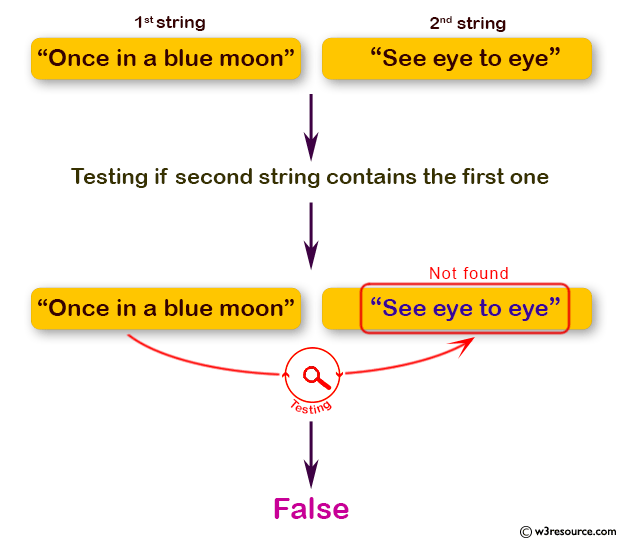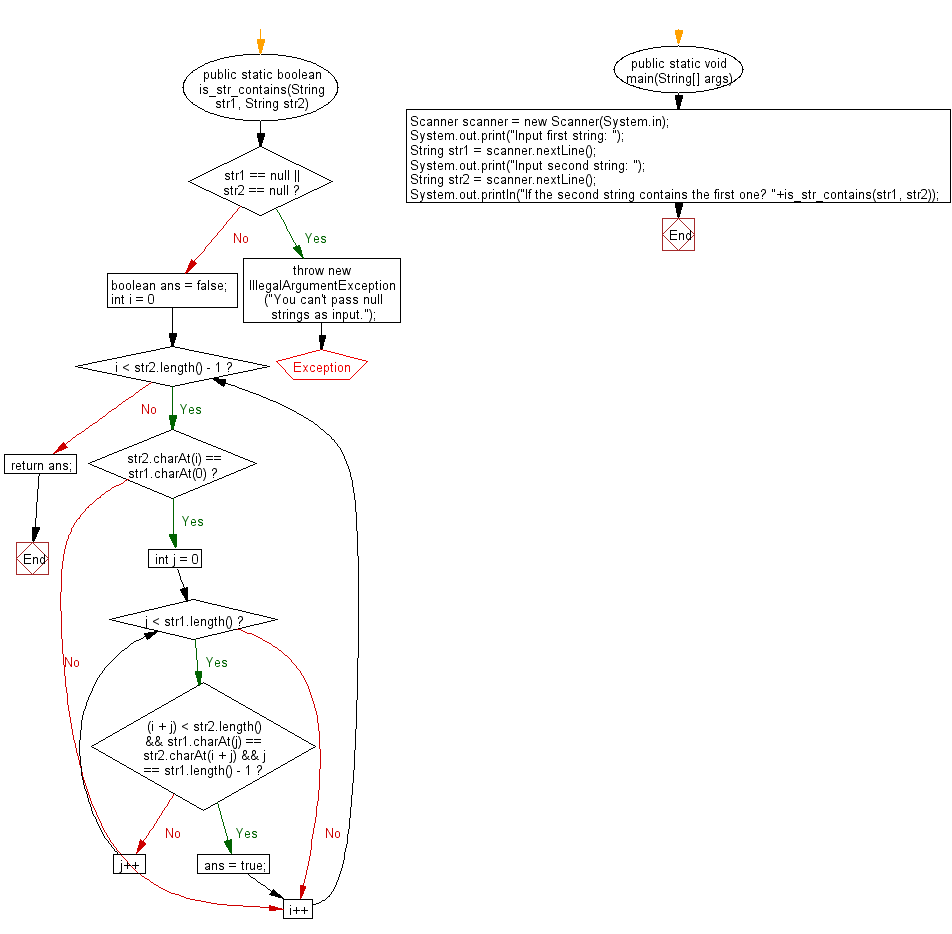﻿ Java programming exercises: Accept two string and test if the second string contains the first one - w3resource# Java Exercises: Accept two string and test if the second string contains the first one

## Java Basic: Exercise-171 with Solution

Write a Java program to accept two string and test if the second string contains the first one.

Pictorial Presentation:Sample Solution:

Java Code:

``````import java.util.*;
public class Solution {
public static boolean is_str_contains(String str1, String str2) {
if (str1 == null || str2 == null) {
throw new IllegalArgumentException("You can't pass null strings as input.");
}
boolean ans = false;
for (int i = 0; i < str2.length() - 1; i++) {
if (str2.charAt(i) == str1.charAt(0)) {
for (int j = 0; j < str1.length(); j++) {
if ((i + j) < str2.length() && str1.charAt(j) == str2.charAt(i + j) && j == str1.length() - 1) {
ans = true;
break;
}
}
}
}
return ans;
}

public static void main(String[] args) {
Scanner scanner = new Scanner(System.in);
System.out.print("Input first string: ");
String str1 = scanner.nextLine();
System.out.print("Input second string: ");
String str2 = scanner.nextLine();
System.out.println("If the second string contains the first one? "+is_str_contains(str1, str2));
}
}
```
```

Sample Output:

```Input first string:  Once in a blue moon
Input second string:  See eye to eye
If the second string contains the first one? false
```

Flowchart:Java Code Editor:

What is the difficulty level of this exercise?

﻿

## Java: Tips of the Day

Directory Content:

Java allows you to get the names of all subdirectories and files in a folder as an array, which can then be sequentially viewed.

```import java.io.*;

public class ListContents {
public static void main(String[] args) {
File file = new File("//home//user//Documents/");
String[] files = file.list();

System.out.println("Listing contents of " + file.getPath());
for(int i=0 ; i < files.length ; i++)
{
System.out.println(files[i]);
}
}
}
```